# (CS 267, Mar 16 1995)

## Introduction to Graph Partitioning

We consider a weighted, undirected graph G=(N, E, W_N, W_E) without self edges (i,i) or multiple edges from one node to another. When we refer to a graph in this lecture, we will always mean this kind of graph. If W_N or W_E is omitted from the definition of G, each weight is assumed to be 1. We use the notation |N| (or |E|) to mean the number of members of the set N (or E).

We think of a node n in N as representing an independent job to do, weight W_n as measuring the cost of job n, and an edge e=(i,j) in E as meaning that an amount of data W_e must be transferred from job i to job j to complete the overall task. Partitioning G, which was introduced in Lecture 16 , means dividing N into the union of P disjoint pieces

```       N = N1 U N2 U ... U NP
```
where the nodes in Ni are assigned to be done by processor Pi. This partitioning is done subject to the optimality conditions below.
1. The sums of the weights W_n of the nodes n in each Ni is approximately equal. This means the load is approximately balanced across processors.
2. The sum of the weights W_e of edges connecting nodes in different Ni and Nj should be minimized. This means that the total size of all messages communicated between different processors is minimized.
This problem arises in a variety of parallel computing problems, such as sparse matrix-vector multipication, and DNA sequencing. We give examples below.

We present a variety of useful algorithms for solving this problem. For simplicity, we will present most of them with unit weights W_n = 1 and W_e = 1, although most can be generalized to general weights.

To illustrate, the following figure shows two partitions of a graph with 8 nodes onto 4 processors, with 2 nodes per processor. The partitioning on the left has 6 edges crossing processor boundaries and so is superior to the partitioning on the right, with 10 edges crossing processor boundaries. The reader is invited to find another 6-edge-crossing partition, and show that no fewer edge crossings suffice.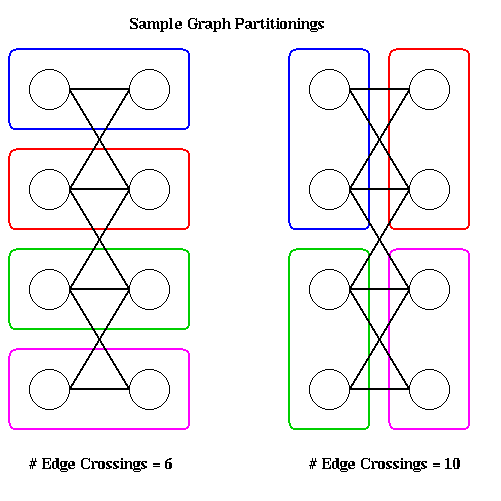In general, computing the optimal partitioning is an NP-complete problem; it is equivalent to the max-cut problem, as we will later describe. Therefore we need to use heuristics to get approximate solutions.

There are two classes of heuristics, depending on the information available about the graph G. In the first case, we have coordinate information with each node, such as (x,y) or (x,y,z) coordinates. This is frequently available if the graph comes from triangulating or otherwise discretizing a physical domain. For example, in the NASA Airfoil, there are (x,y) coordinates associated with each node (to see the data and be able to manipulate it, start up matlab and type "airfoil"). Typically, such graphs have the additional property that only nodes which are spatially close together have edges connecting them. We may use this property to develop two efficient geometrical algorithms for graph partitioning.

The second kind of graph we want to partition is coordinate free, i.e. we have no identification of a node with a physical point in space. In addition, edges have no simple interpretation such a representing physical proximity. These kinds of graphs require other algorithms to be used (which may obviously also be applied to graphs with coordinate information).

Almost all the algorithms we discuss are only for graph bisection, i.e. they partition N = N1 U N2. They are intended to be applied recurively, bisecting N1 and N2, etc, until the partitions are small and numerous enough.

## Edge Separators and Vertex Separators

Bisecting a graph G=(N,E) can be done in two ways. In the last section, we discussed finding the smallest subset Es of E such that removing Es from E divided G into two disconnected subgraphs G1 and G2, with nodes N1 and N2 respectively, where N1 U N2 = N and N1 and N2 are disjoint and equally large. The edges in Es connect nodes in N1 to nodes in N2. Since removing Es disconnects G, Es is called an edge separator.

The other way to bisect a graph is to find a vertex separator, a subset Ns of N, such that removing Ns and all incident edges from G also results in two disconnected subgraphs G1 and G2 of G. In other words N = N1 U Ns U N2, where all three subsets of N are disjoint, N1 and N2 are equally large, and no edges connect N1 and N2.

The following figure illustrates these ideas. The green edges, Es1, form an edge separator, as well as the blue edges Es2. The red nodes, Ns, are a vertex separator, since removing them and the indicident edges (Es1, Es2, and the purple edges), leaves two disjoint subgraphs.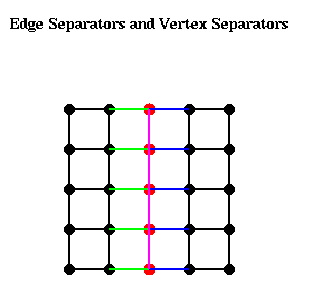Given an edge separator Es, one can produce a vertex separator Ns by taking one end-point of each edge in Es; Ns clearly has at most as many members as Es. Given a vertex separator Ns, on can produce an edge separator Es by taking all edges incident to Ns; Es clearly has at most d times as many members as Es, where d is the maximum degree (number of incident edges) of any node. Thus, if d is small, finding good edge separators and good vertex separators are closely related problems, a fact we exploit below.

## Partitioning Graphs with Coordinate Information

### Partitioning Planar Graphs

We begin with a theorem about partitioning planar graphs, i.e. graphs G=(N,E) which can be drawn in the plane without crossing edges. Such graphs include the NASA Airfoil, and similar triangulations of two dimensional objects. We consider the problem of finding a vertex separator Ns. To get some intuition, consider the very simple m-by-m grid of nodes, where each node is connected to its neighbors to the north, south, east and west (the 5-by-5 case is shown above). Clearly, the graph can be separated by removing m nodes along a column or row in the middle of the matrix. In other words,
```   |S| = m = sqrt(m^2) = sqrt(number of nodes).
```
It turns out that it is generally possible to find a vertex separtor containing about the square root of the number of nodes.

Theorem. (Tarjan, Lipton, "A separator theorem for planar graphs", SIAM J. Appl. Math., 36:177-189, April 1979). Let G=(N,E) be an planar graph. Then we can find a vertex separator Ns, so that N = N1 U Ns U N2 is a disjoint partition of N, |N1| <= (2/3)*|N|, |N2| <= (2/3)*|N|, and |Ns| <= sqrt(8*|N|).

In other words, there is a vertex separator Ns that separate the nodes N into two parts, N1 and N2, neither of which can be more than twice as large as the other, and Ns has more more than about the square root of the number of nodes. This intuition motivates the algorithms below.

### Inertial Partitioning

This graph bisection algorithm is very simple: For a graph with 2D coordinates, it chooses a line such that half the nodes are on one side of the line, and half are on the other. For a graph with 3D coordinates, it chooses a plane with the same property. This can be done as follows (we state the algorithm in two dimensions, since it is essentially the same independent of the number of dimensions).
1. Choose a straight line L, given by a*(x-xbar)+b(y-ybar) = 0. This is a straight line through (xbar,ybar), with slope -a/b. We assume without loss of generality that a^2 + b^2 = 1.
2. For each node ni=(xi,yi), compute a coordinate by computing the dot-product Si = -b*(xi-xbar) + a*(yi-ybar). Si is distance from (xbar,ybar) of the projection of (xi,yi) onto the line L.
3. Find the median value Sbar of the Si's.
4. Let the nodes (xi,yi) satisfying Si <= Sbar be in partition N1, and the nodes where Si > Sbar be in partition N2.
This is shown in the figure below. No edges are shown, since these are not used by the algorithm, although it is clear that if nearest neighbors only are connected, then very few edges will cross the blue dotted line separating N1 and N2. Clearly the algorithm can be implemented in time O(|N|).It remains to show how to pick the line L. Intuitively, if the nodes are located in a long, thin region of the plane, as in the above example, we want to pick L along the long axis this region: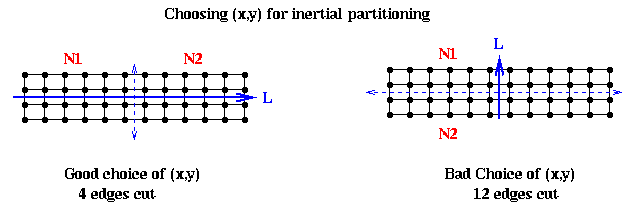In mathematical terms, we want to pick a line such that the sum of squares of lengths of the green lines in the figure are minimized; this is also called doing a total least squares fit of a line to the nodes. In physical terms, if we think of the nodes as unit masses, we choose (x,y) to be the axis about which the moment of inertia of the nodes is minimized. This is why the method is called inertial partitioning. This means choosing a, b, xbar and ybar so that a^2 + b^2 = 1, and the following quantity is minimized:

```     sum_{i=1}^{|N|} (length of i-th green line)^2
= sum_{i=1}^{|N|} ((xi-xbar)^2 + (yi-ybar)^2 - (-b*(xi-xbar) + a*(yi-ybar))^2 )
... by the Pythagorean theorem
=   a^2 *   ( sum_{i=1}^{|N|} (xi-xbar)^2 )
+ b^2 *   ( sum_{i=1}^{|N|} (yi-ybar)^2 )
+ 2*a*b * ( sum_{i=1}^{|N|} (xi-xbar)*(yi-ybar) )
= a^2 * X2 + b^2 * Y2 + 2*a*b * XY
= [ a b ] * [ X2  XY ] * [ a ]
[ XY  Y2 ]   [ b ]
= [ a b ] * M * [ a ]
[ b ]
```
where X2, Y2 and XY are the summations in the previous lines. On can show that an answer is to choose
```   xbar = sum_{i=1}^{|N|} xi / |N|
ybar = sum_{i=1}^{|N|} yi / |N|
```
i.e. (xbar,ybar) is the "center of mass" of the nodes, and (a,b) is the unit eigevector corresponding to the smallest eigenvalue of the matrix M.

The 3D (and higher) case is similar, except that we get a 3-by-3 matrix whose eigenvector we need.

### Partitioning with Random Circles

The Lipton-Tarjan planar graph separator theorem provides intuition for the success of inertial graph partitioning: One hopes to cut just O(sqrt(|N|) edges , a small fraction of the total number of edges |E| = Omega (|N|). In 3 and higher dimensions, we might hope to do simiarly well, at least for graphs similar to grids. Consider an n-by-n-by-n grid with |N|=n^3 nodes, each node connected to its neighbors along grid lines. By taking the edges intersecting a plane parallel to two of the axes and bisecting the cube, one can find an edge separator with n^2 = N^(2/3) = O(|E|^(2/3)) edges. Similarly, a d-dimensional grid with n^d nodes has a separator with O(|E|^((d-1)/d)) edges.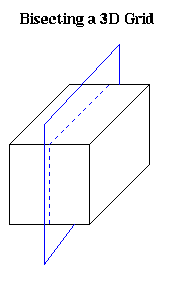To extend our intuition about planar graphs to higher dimensions, we need a notion of graphs that are well-shaped in some sense, so that the cells created by edges joining nodes (triangles in 2D, simplices in 3D, etc.) cannot have angles that are too large or too small. The following approach is taken by G. Miller, S.-H. Teng, W. Thurston and S. Vavasis ("Automatic mesh partitioning", in Graph Theory and Sparse Matrix Computation, Springer-Verlag 1993, editted by J. George, J. Gilbert and J. Liu). Our presentation is drawn from "Geoemtric Mesh Paritioning" by J. Gilbert, G. Miller and S.-H. Teng (available from gilbert@parc.xerox.com).

Definition. A k-ply neighborhood system in d dimensions is a set {D1,...,Dn} of closed disks in d-dimensional real space R^d, such that no point in R^d is strictly interior to more than k disks.

Definition. An (alpha,k) overlap graph is a graph defined in terms of a constant alpha >= 1, and a k-ply neighborhood system {D1,...,Dn}. There is a node for each disk Di. There is an edge (i,j) if expanding the radius of the smaller of Di and Dj by a factor alpha causes the two disks to overlap. For example, an n-by-n mesh is a (1,1) overlap graph, as shown below. The same is true for any d-dimensional grid. One can show any planar graph is an (alpha,k) overlap graph.One can prove the following generalization of the Lipton-Tarjan theorem:

Theorem. (Miller, Teng, Thurston, Vavasis). Let G=(N,E) be an (alpha,k) overlap graph in d dimensions with n nodes. Then there is a vertex separator Ns, so that N = N1 U Ns U N2, and

1. N1 and N2 each has at most (d+1)/(d+2)*n nodes, and
2. Ns has at most O(alpha * k^(1/d) * n^((d-1)/d) ) nodes.
When d=2, the bounds on the sizes of N1, N2 and Ns are just those given by Lipton and Tarjan.

The separator is constructed by choosing a sphere in d-dimensional space, using the edges it cuts as an edge separator, and extracting the corresponding vertex separator. There is a randomized algorithm for choosing the sphere from a particular probability distribution such that it satisfies the conditions of the theorem with high probability.

To describe the algorithm, we need to explain stereographic projection, which is a way of mapping points in a d-dimensional space to the unit sphere centered at the origin in (d+1) dimensional space. When d=2, this is done by drawing a line from the point p to the north pole of the sphere; the point p' where the line and sphere intersect is the stereographic projection of p. The same idea applies to higher dimensions.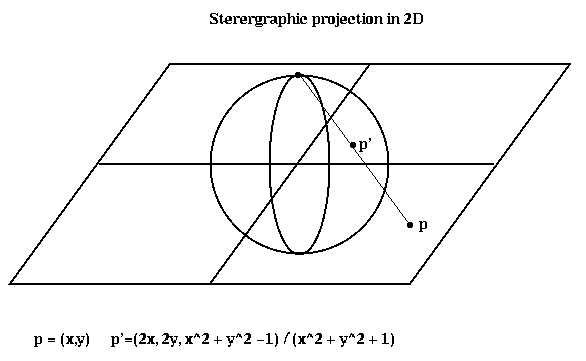Here is the algorithm. Matlab software implementing it is available via anonymous ftp from ftp.parc.xerox.com at /pub/gilbert/meshpart.uu.

1. Project up. Stereographically project the nodes of the graph in d-dimensions to the unit sphere in d+1 dimensions.
2. Find the centerpoint of the projected points. The centerpoint has the property that every hyperplane through the centerpoint divides the points into two approximately even subsets (in the ratio d:1 or better). A centerpoint can be found by linear programming, but cheaper heurstics exist. See the paper by Miller, Gilbert and Teng for details.
3. Conformally map the points on the sphere. This is done in two steps. First, rotate the projected points around the origin, including the centerpoint, so that the centerpoint has coordinates (0,...,0,r), for some r. Second, dilate the points. This is done by reversing the stereographic projection (projecting the rotated points on the sphere back to the plane), multiplying their coordinates in the plane by sqrt((1-r)*(1+r)), and sterographically projecting back to the sphere. One can show that the new centerpoint of the conformally mapped points lies at the origin of the sphere.
4. Pick a random d-dimensional plane though the centerpoint (origin of the sphere). The intersectio of this plane and the sphere is a circle. This circle is the sterographic image of another circle C in d-dimensional space.
5. Convert the circle C to a vertex separator. A node i belongs to Ns if its disk Di, with radius magnified by alpha, intersects C.
Miller et al show that the average separator formed by choosing a random circle this way satisfies the conditions of the theorem, so if one chooses a few random circles, with high probability this one of the them will form a good separator.